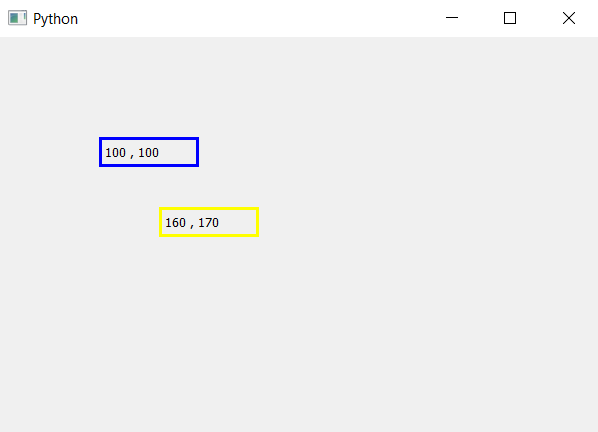Open in App
Not now

# PyQt5 – How to get Label coordinates ?

• Last Updated : 26 Mar, 2020

The main window in PyQt5 is like graph it has x-axis and y-axis, all the widgets are positioned according to their x, y co-ordinates. When we create label is formed at the top left corner, the op left co-ordinates are (0, 0), we can move the label using `move()` method.
In this article, we will see how to get the co-ordinates of label. In order to do so we will use `x()` method for x co-ordinate and `y()` method for y co-ordinate.

Syntax :

```x_position = label.x()
y_position =label.y()
```

Argument : Both method takes no argument.

Return : Both returns integer.

Code :

 `# importing the required libraries`` ` `from` `PyQt5.QtCore ``import` `*` `from` `PyQt5.QtGui ``import` `*` `from` `PyQt5.QtWidgets ``import` `*` `import` `sys`` ` ` ` `class` `Window(QMainWindow):`` ` ` ` `    ``def` `__init__(``self``):``        ``super``().__init__()`` ` ` ` `        ``# set the title``        ``self``.setWindowTitle(``"Python"``)`` ` `        ``# setting  the geometry of window``        ``self``.setGeometry(``60``, ``60``, ``600``, ``400``)`` ` ` ` `        ``# creating a label widget``        ``self``.label_1 ``=` `QLabel(``self``)`` ` `        ``# moving position``        ``self``.label_1.move(``100``, ``100``)`` ` `        ``# setting up the border``        ``self``.label_1.setStyleSheet(``"border :3px solid blue;"``)`` ` `        ``# getting x and y co-ordinates``        ``x ``=` `str``(``self``.label_1.x())``        ``y ``=` `str``(``self``.label_1.y())`` ` `        ``# setting label text``        ``self``.label_1.setText(x``+``", "``+` `y)`` ` `        ``# creating a label widget``        ``self``.label_2 ``=` `QLabel(``self``)`` ` `        ``# moving position``        ``self``.label_2.move(``160``, ``170``)`` ` `        ``# setting up the border``        ``self``.label_2.setStyleSheet(``"border :3px solid yellow;"``)`` ` `        ``# getting x and y co-ordinates``        ``x ``=` `str``(``self``.label_2.x())``        ``y ``=` `str``(``self``.label_2.y())`` ` `        ``# setting label text``        ``self``.label_2.setText(x ``+` `", "` `+` `y)`` ` `        ``# show all the widgets``        ``self``.show()`` ` ` ` `# create pyqt5 app``App ``=` `QApplication(sys.argv)`` ` `# create the instance of our Window``window ``=` `Window()``# start the app``sys.exit(App.``exec``())`

Output :My Personal Notes arrow_drop_up